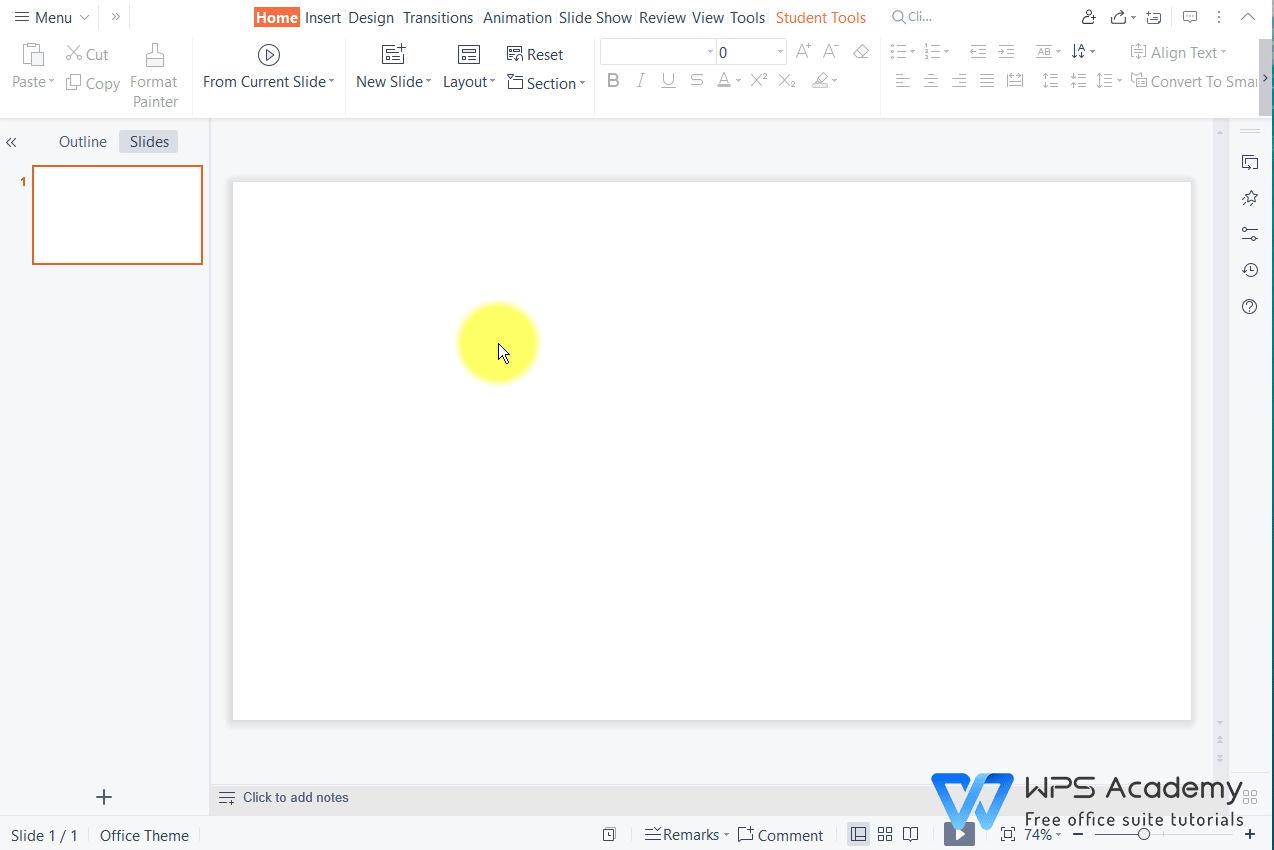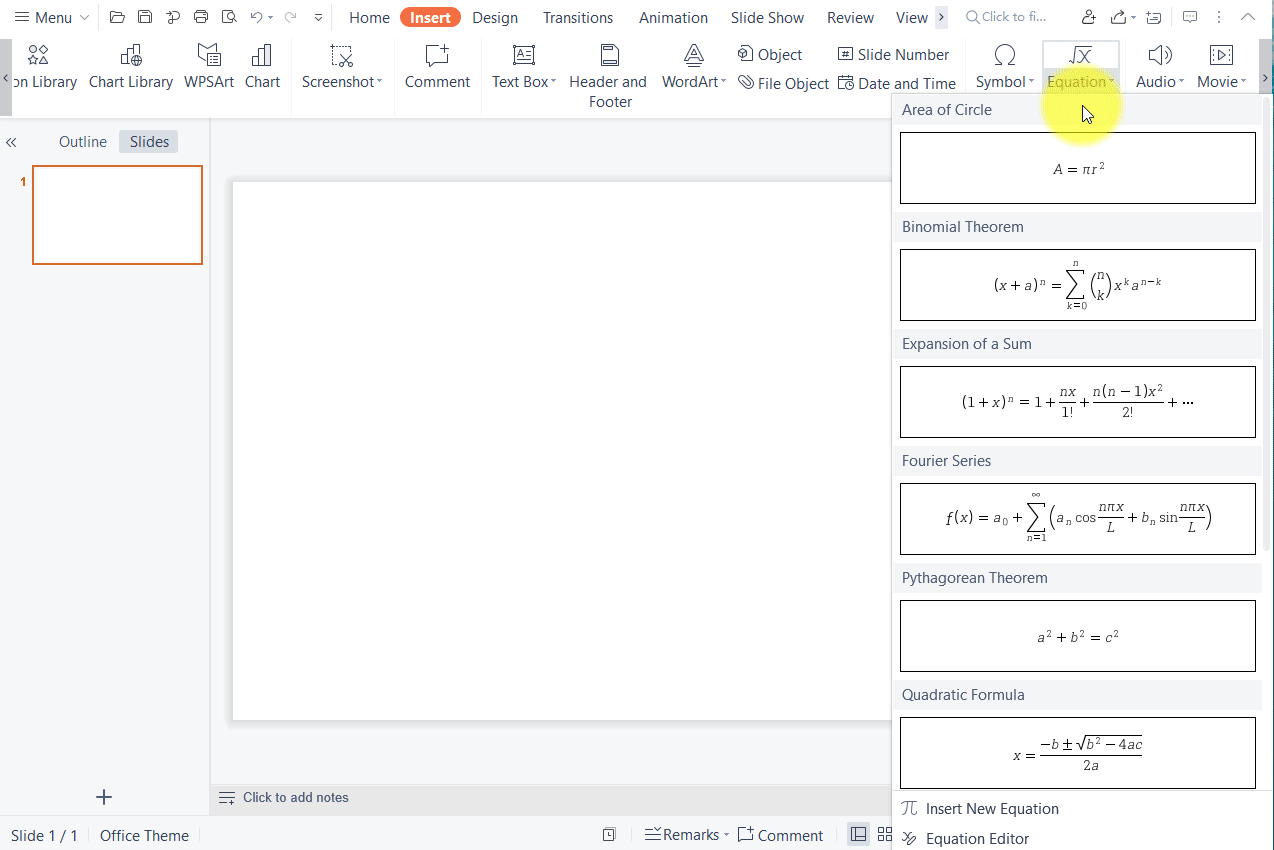How to enter exponent in PowerPoint?

January 6, 2022
82 Views

Graphic skills

WPS Presentation supports entering mathematical equations quickly. Here are the steps to enter exponent.

1. Click the Insert tab > the Equation drop-down button.

2. Here are several basic equations for quick application. We can choose any of them and edit it as needed.3. If you need more, click Equation EditorEnter your equation in the editing area, when you need to add exponent, click Subscript and Superscript templates > Superscript. Then enter the needed value in the box.With Equation Editor, you can add any complex equations you need quickly and freely. Try to explore it!